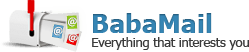# Math Quiz: What is the Logic Behind These Equations?

A+ A-

To pass this math quiz, you don't need to be a math wiz. You just need to be able to figure out the rules governing the following equations and then complete the last one. Some may seem tough, but once you find the rule behind each equation it should be easy.